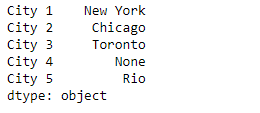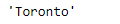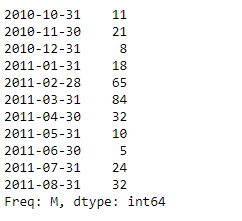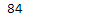# Python | Pandas Series.get()

Pandas series is a One-dimensional ndarray with axis labels. The labels need not be unique but must be a hashable type. The object supports both integer- and label-based indexing and provides a host of methods for performing operations involving the index.

Pandas` Series.get()` function get item from object for given key (DataFrame column, Panel slice, etc.). Returns default value if not found.

Syntax: Series.get(key, default=None)

Parameter :
key : object

Returns : value : same type as items contained in object

Example #1: Use `Series.get()` function to get the value for the passed index label in the given series object.

 `# importing pandas as pd ` `import` `pandas as pd ` ` `  `# Creating the Series ` `sr ``=` `pd.Series([``'New York'``, ``'Chicago'``, ``'Toronto'``, ``None``, ``'Rio'``]) ` ` `  `# Create the Index ` `index_ ``=` `[``'City 1'``, ``'City 2'``, ``'City 3'``, ``'City 4'``, ``'City 5'``]  ` ` `  `# set the index ` `sr.index ``=` `index_ ` ` `  `# Print the series ` `print``(sr) `

Output :Now we will use `Series.get()` function to return the value for the passed index label in the given series object.

 `# return the value corresponding to ` `# the passe index label ` `result ``=` `sr.get(key ``=` `'City 3'``) ` ` `  `# Print the result ` `print``(result) `

Output :As we can see in the output, the `Series.get()` function has returned the value corresponding to the passed index label.

Example #2 : Use `Series.get()` function to get the value for the passed index label in the given series object.

 `# importing pandas as pd ` `import` `pandas as pd ` ` `  `# Creating the Series ` `sr ``=` `pd.Series([``11``, ``21``, ``8``, ``18``, ``65``, ``84``, ``32``, ``10``, ``5``, ``24``, ``32``]) ` ` `  `# Create the Index ` `index_ ``=` `pd.date_range(``'2010-10-09'``, periods ``=` `11``, freq ``=``'M'``) ` ` `  `# set the index ` `sr.index ``=` `index_ ` ` `  `# Print the series ` `print``(sr) `

Output :Now we will use `Series.get()` function to return the value for the passed index label in the given series object.

 `# return the value corresponding to ` `# the passe index label ` `result ``=` `sr.get(key ``=` `'2011-03-31'``) ` ` `  `# Print the result ` `print``(result) `

Output :As we can see in the output, the `Series.get()` function has returned the value corresponding to the passed index label.

My Personal Notes arrow_drop_upCheck out this Author's contributed articles.

If you like GeeksforGeeks and would like to contribute, you can also write an article using contribute.geeksforgeeks.org or mail your article to contribute@geeksforgeeks.org. See your article appearing on the GeeksforGeeks main page and help other Geeks.

Please Improve this article if you find anything incorrect by clicking on the "Improve Article" button below.

Article Tags :

Be the First to upvote.

Please write to us at contribute@geeksforgeeks.org to report any issue with the above content.